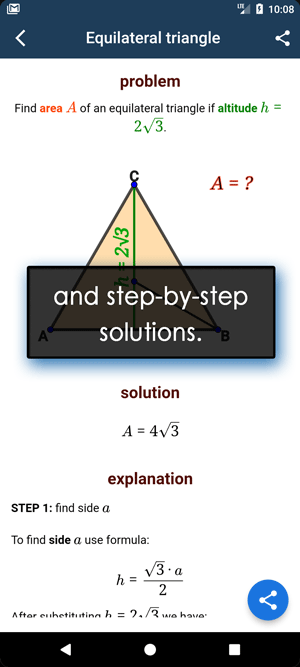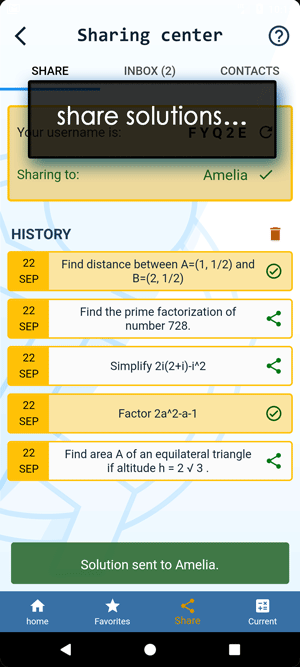Math Calculators, Lessons and Formulas

It is time to solve your math problem

mathportal.org

# Polynomial graphing calculator

This page helps you explore polynomials with degrees up to 4. The roots (x-intercepts), signs, local maxima and minima, increasing and decreasing intervals, points of inflection, and concave up-and-down intervals can all be calculated and graphed.

Polynomial Graphing Calculator
Explore and graph polynomials.
show help ↓↓ examples ↓↓
x^2+3x-4
2x^3-3x^2-2x+3
x^4/12-x^3/3-7x^2/12+5x/6working...
examples
example 1:ex 1:
Sketch the graph of polynomial $p(x) = x^3-2x^2-24x$
example 2:ex 2:
Find relative extrema of a function $f(x) = x^3-x$
example 3:ex 3:
Find the inflection points of $-x^4+x^2+4$
example 4:ex 4:
Sketch the graph of polynomial $p(x) = x^4-2x^2-3x+4$
Search our database of more than 200 calculators